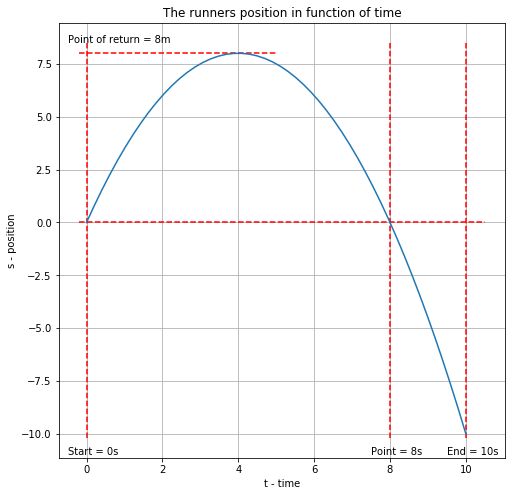Chapter-2 Q030 - Physics For Engineers And Scientists - SolutionsON7AMI Amateur Radio, Meteology and Astronomy website. JO10UX# Question 30 - Problems - Chapter 2¶

## Problem:¶

The position of a person is given by s = 4.0t - 0.50t^2, where X is in meters and t is in seconds.

## Question:¶

a) What is the average velocity between t=0 and t=8.0 s?

b) And between t=8.0 s and t=10.0 s?

## Solution:¶

The position of the person is given by a parabolic position.

The factor of $t¨2$ is negative so the top of the parabol will be up.

The top (or maximal distance is given by the first derivative:

$$\frac{dx}{dt}=\frac{d}{dt}(-0.5t^2+4t)$$

We find the turn aroud point by solving for speed = 0

$$\implies-0.5x2t+4=0$$

$$\implies t=\frac{-4}{-1}$$

$$\implies t = 4$$

The average velocity:

$$\overline v=\frac{\bigtriangleup s}{\bigtriangleup t}$$

The path in function of the time is described in the figure below:

In :
from sympy import *
import numpy as np
import matplotlib.pyplot as plt
t_val = np.linspace(0,10,50)
s_val = 4*t_val - 0.5*t_val**2
plt.figure(figsize=(8,8))
plt.plot(t_val, s_val)
plt.grid()
plt.title('The runners position in function of time')
plt.xlabel('t - time')
plt.ylabel('s - position')
plt.hlines(y=0, xmin=-0.2, xmax=10.5, color='r', linestyles='dashed')
plt.hlines(y=8, xmin=-0.2, xmax=5, color='r', linestyles='dashed')
plt.annotate('Point of return = 8m', xy=(-0.5, 8.5))
plt.vlines(x=0, ymin=-10.2, ymax=8.5, color='r', linestyles='dashed')
plt.vlines(x=8, ymin=-10.2, ymax=8.5, color='r', linestyles='dashed')
plt.vlines(x=10, ymin=-10.2, ymax=8.5, color='r', linestyles='dashed')
plt.annotate('Start = 0s', xy=(-0.5, -11))
plt.annotate('Point = 8s', xy=(7.5, -11))
plt.annotate('End = 10s', xy=(9.5, -11))
plt.show()Displacement $\Delta d_{0-8s}= 8 + (- 8) = 0m$

Traveled way $s_{0-8s} = |8| + |-8| = 16m$

Displacement $\Delta d_{8-10s}= -10m$

Traveled way $s_{8-10s} = |-10| = 10m$

In :
t_8 = 8
t_10 = 2
d_8 = 0
s_8 = 16
d_10 = -10
s_10 = 10
v_v_8 = d_8/t_8
v_s_8 = s_8/t_8
v_v_10 = d_10/t_10
v_s_10 = s_10/t_10
print(f'a) The average velocity between 0s and 8s is: {v_v_8:.2f} m/s')
print(f'b) The average velocity between 8s and 10s is: {v_v_10:.2f} m/s')
print('')
print(f'- The average speed of the first part is {v_s_8:.2f} m/s an of the second part is {v_s_10:.2f} m/s')

a) The average velocity between 0s and 8s is: 0.00 m/s
b) The average velocity between 8s and 10s is: -5.00 m/s

- The average speed of the first part is 2.00 m/s an of the second part is 5.00 m/s


 سُوۡرَةُ حٰمٓ السجدة / فُصّلَت ثُمَّ اسْتَوَىٰ إِلَى السَّمَاءِ وَهِيَ دُخَانٌ فَقَالَ لَهَا وَلِلْأَرْضِ ائْتِيَا طَوْعًا أَوْ كَرْهًا قَالَتَا أَتَيْنَا طَائِعِينَ ﴿١١ فَقَضَاهُنَّ سَبْعَ سَمَاوَاتٍ فِي يَوْمَيْنِ وَأَوْحَىٰ فِي كُلِّ سَمَاءٍ أَمْرَهَا ۚ وَزَيَّنَّا السَّمَاءَ الدُّنْيَا بِمَصَابِيحَ وَحِفْظًا ۚ ذَٰلِكَ تَقْدِيرُ الْعَزِيزِ الْعَلِيمِ ﴿١٢ Surah Fussilat: Thereafter turned He to the heaven and it was as smoke, and said Unto it and Unto the earth: come ye twain, willingly or loth. They said: we come willingly. (11) Then He decreed them as seven heavens in two days, and revealed Unto each heaven the command thereof; and We bedecked the nether heaven with lamps and placed therein a guard. That is the ordinance of the Mighty, the Knower. (12) De hemelen en aarde (waren) een samenhangende massa. Wij hebben ze toen van elkaar gescheiden… Wij hebben de hemel tot een beschermend dak gemaakt … en de dag en de nacht, de zon en de maan geschapen. (Koran 41 11:12) Copyright (c) 2000-2020 - Jean Paul Mertens - ON7AMI - formal ON1AMI - Grote Steenweg 86 - 9840 Zevergem - Belgium - Europe